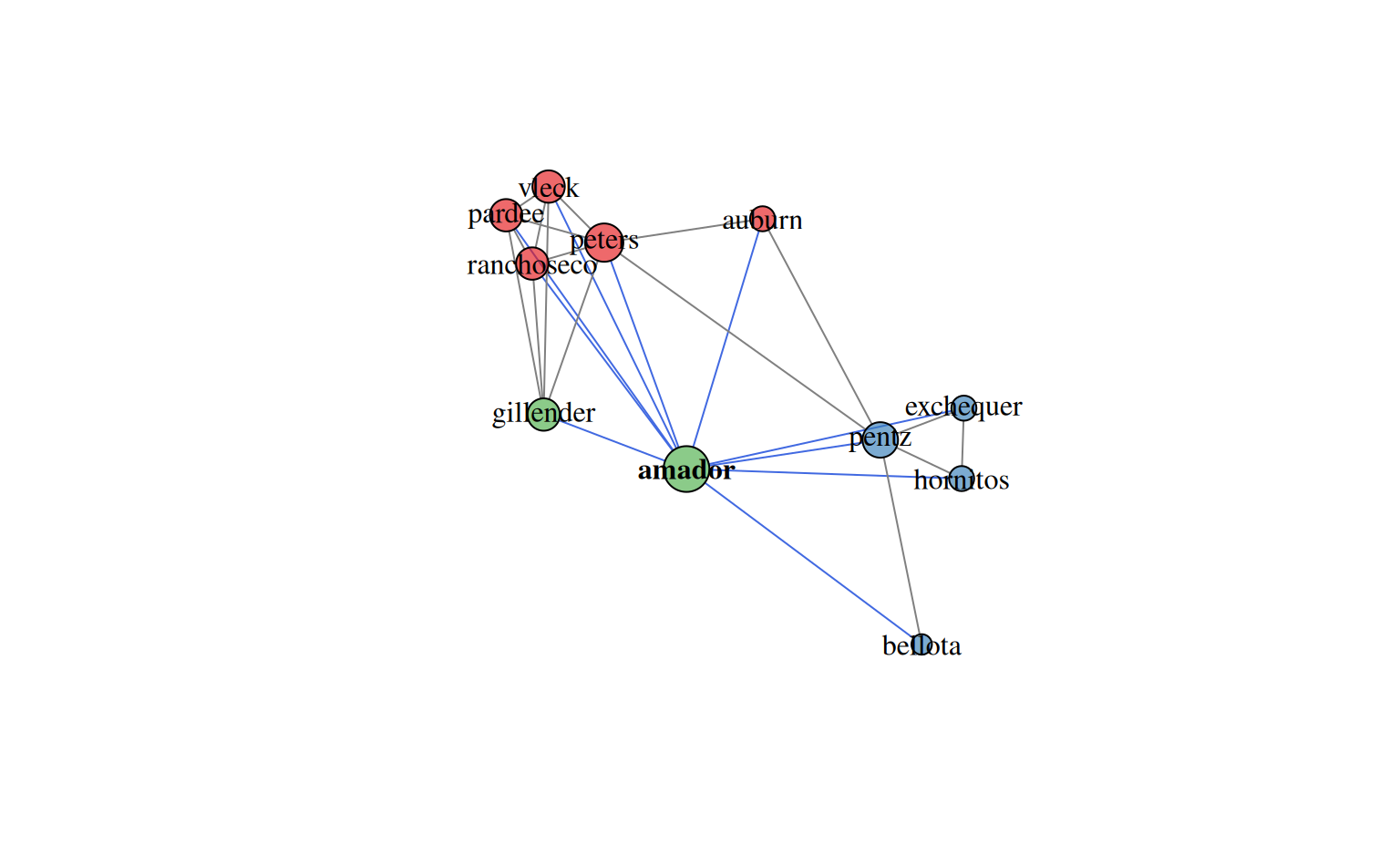Create an adjacency matrix from SSURGO component data

component.adj.matrix(
d,
mu = "mukey",
co = "compname",
wt = "comppct_r",
method = c("community.matrix", "occurrence"),
standardization = "max",
metric = "jaccard",
rm.orphans = TRUE,
similarity = TRUE,
return.comm.matrix = FALSE
)

## Arguments

d

data.frame, typically of SSURGO data

mu

name of the column containing the map unit ID (typically 'mukey')

co

name of the column containing the component ID (typically 'compname')

wt

name of the column containing the component weight percent (typically 'comppct_r')

method

one of either: community.matrix, or occurrence; see details

standardization

community matrix standardization method, passed to vegan::decostand

metric

community matrix dissimilarity metric, passed to vegan::vegdist

rm.orphans

logical, should map units with a single component be omitted? (typically yes)

similarity

logical, return a similarity matrix? (if FALSE, a distance matrix is returned)

return.comm.matrix

logical, return pseudo-community matrix? (if TRUE no adjacency matrix is created)

## Value

a similarity matrix / adjacency matrix suitable for use with igraph functions or anything else that can accommodate a similarity matrix.

D.E. Beaudette

## Examples

if (requireNamespace("igraph")) {